# Slope Fields

In this section, we'll see the first method we have of analyzing differential equations that we don't know how to solve. This method will work for any first-order differential equation. We'll start by learning how to draw a picture that represents all the possible solutions to a first order equation, but we'll quickly see that while it's not that difficult to do, it can be very tedious, so we generally rely on computers to draw these graphs for us. The skill we really need, therefore, is the ability to read these graphs and make sense of them.

# Drawing Slope Fields

We'll illustrate this with a simple example: $y' = t + y$

Clearly, $t$ is the independent variable, and $y$ is a function of $t.$ We'll learn in a few sections how to solve this kind of equation, but for now we can't get an explicit solution. However, if we knew the value of $y$ for some value of $t,$ we would also know the value of $y'$ at that point. We can therefore find the value of $y'$ for every possible combination of $t$ and $y.$ Each combination of $t$ and $y$ represents a point that we can plot, and $y'$at that point is the slope of the solution that would pass through that point.

Therefore we can build a table like the one below: $\begin{array}{c c c} t & y & y'=t+y\ \hline 0 & 0 & 0\ 0 & 1 & 1\ 1 & 0 & 1\ 1 & 1 & 2\ \vdots & \vdots & \vdots \end{array}$

To draw the slope field, we sketch a short segment at each point with the appropriate slope. The completed graph looks like the following: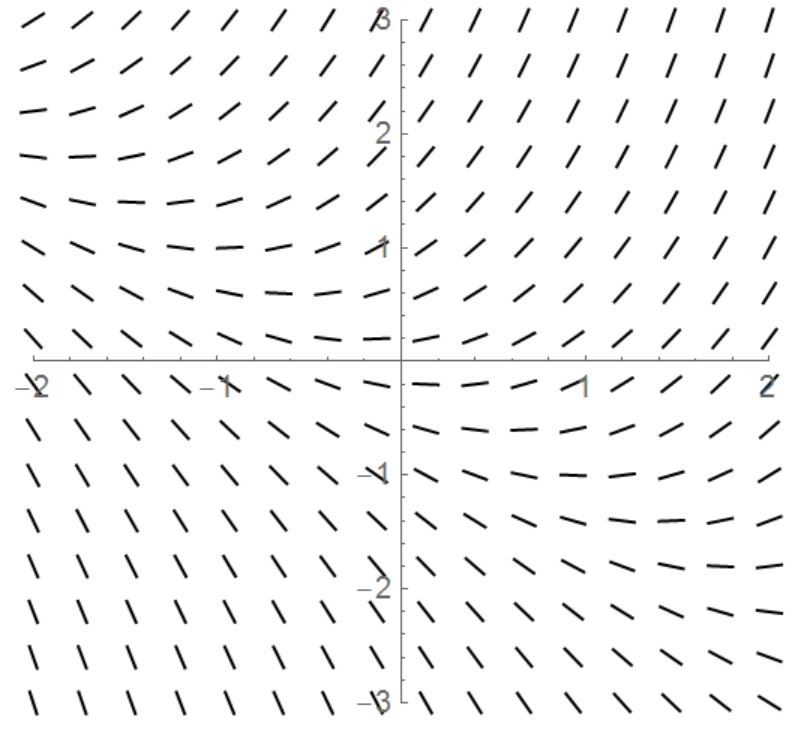## What does a slope field mean?

The most basic way to read a slope field is to think of it as a wind map. If you drop a leaf onto this map, where will it go? This of course depends on where you drop it. The slope field represents all the solutions to the differential equation (the family of solutions we saw at the end of the last section). The specific solution depends on the initial condition, which is the spot where the metaphorical leaf is dropped.

For instance, if the initial condition is $y(0)=1,$ meaning that $y=1$ when $x=0,$ the solution looks like this curve: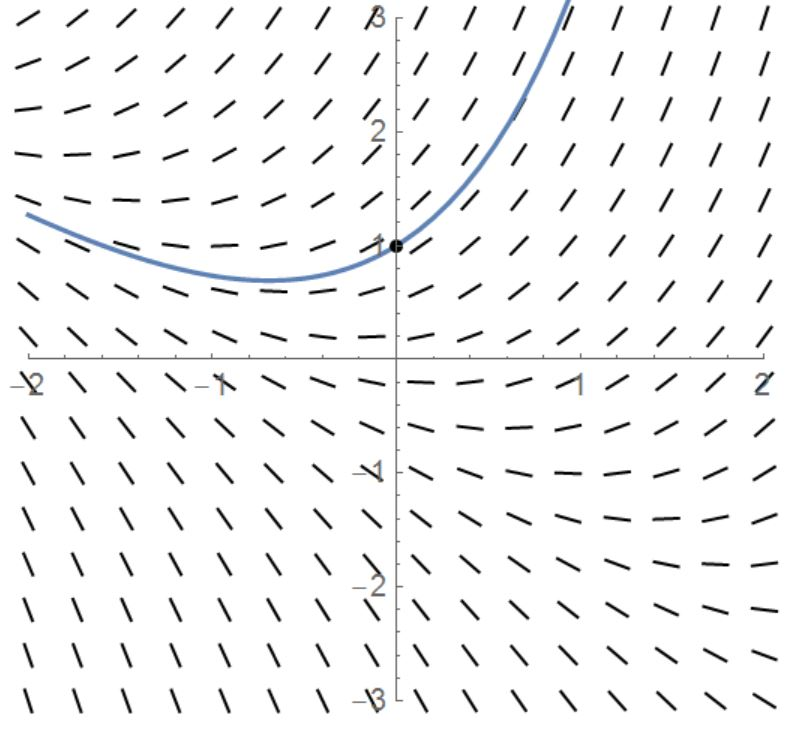If, on the other hand, the initial condition is $y(0)=−2,$ the solution looks like this: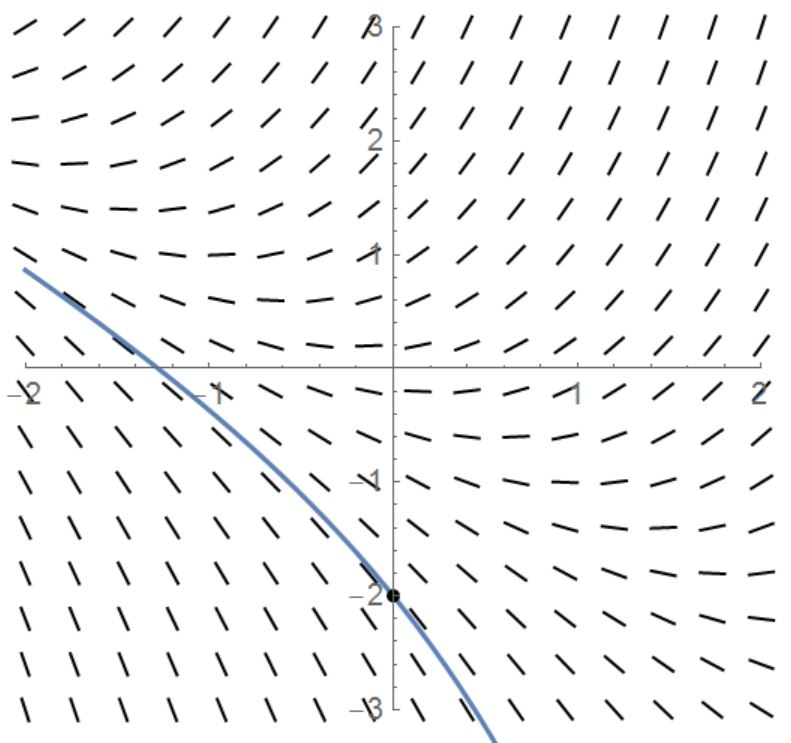# Analyzing Slope Fields

Again, it gets really tedious really fast to draw slope fields by hand, so we use computers to draw them instead. What we need to be able to do is read slope fields, so we'll practice making some simple observations. To do this, I'll use a Wolfram Demonstration, but it may not work with your browser (last time I checked, it worked with Firefox). If you're using Chrome, you should still be able to download the Wolfram CDF player and download the demonstration. Just in case, I'll post screenshots of the relevant parts.

The demonstration is Slope Fields from the Wolfram Demonstrations Project by Charles E. Oelsner. There's also a Desmos calculator project that can draw the same figures.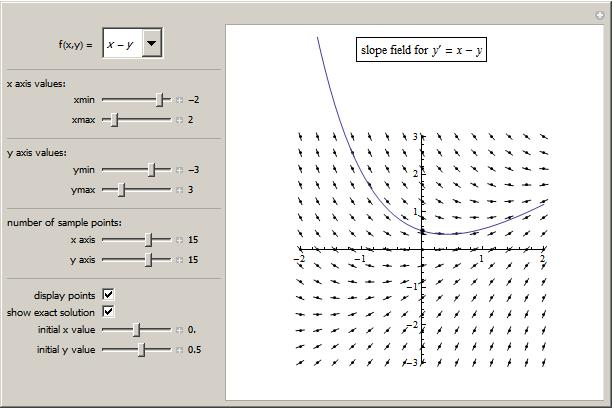Take a look at the following example; we'll start with this one.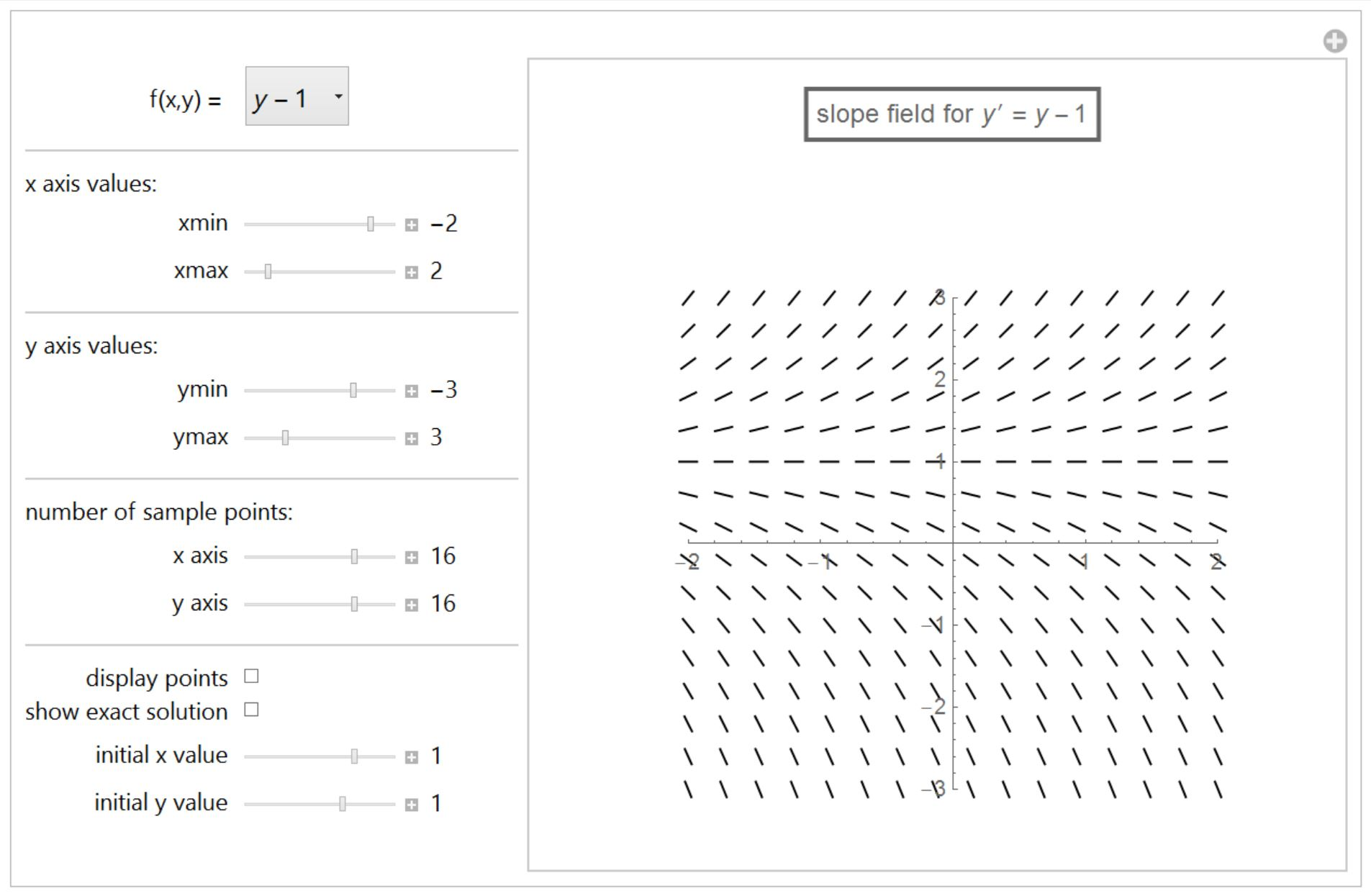We'll make two observations from this slope field:

1. The slopes depend solely on $y$ (we can also tell this simply by looking at the differential equation). Notice that if you look along a horizontal line (where $y$ stays constant), the slopes are all the same. This is called an autonomous differential equation, because it does not depend on the independent variable.
2. More importantly, notice that when $y=1,$ the slopes are all $0.$ This is called an equilibrium solution, because if the initial condition falls on this line, the solution will stay constant for ever.

## Equilibrium Solutions

Equilibrium solutions are one of the most important features that we can observe on a slope field. Look at another example: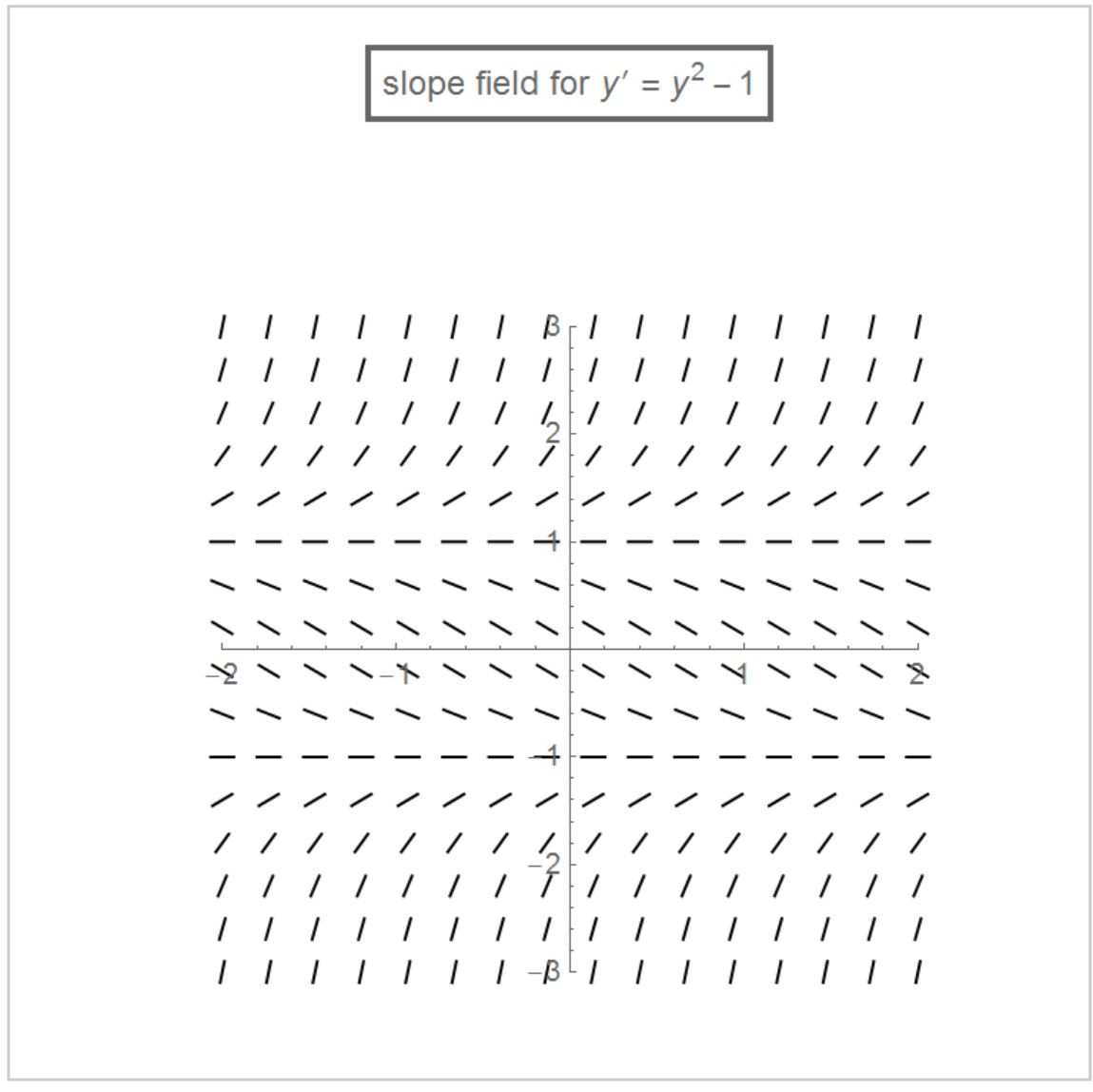You should notice two equilibrium solutions, one at $y=1$ and one at $y=−1$ (we could also have found this by solving $y^2−1=0$). Look at them again, though; there is a distinct difference between them. Around $y=1,$ the slopes diverge from the equilibrium, and around $y=−1$ the slopes converge toward the equilibrium. The top one is called an unstable equilibrium, and the bottom one is is called a stable equilibrium. The two classic ways to illustrate the difference are shown in the following images:The two diagrams on top represent a stable equilibrium. If you place a marble in the bottom of a bowl, it will stay there, and if you start a pendulum in the vertical position, it will stay there as well. This is simply what it means to be an equilibrium; the system doesn't try to change anything. However, these are both stable equilibria because, if you disturb the system slightly (by moving the marble a little bit or by giving the pendulum a small push), it will return to this equilibrium.

On the other hand, consider the two diagrams on the bottom; these represent unstable equilibria. If the bowl is turned upside down, the bottom (now the top) is still an equilibrium point, because if you balance a marble perfectly on the peak, it will stay there. Similarly, with a pendulum pinned at the bottom, if you balance it perfectly, it will not move. However, in either of these cases, if you disturb the system even slightly, it will leave the equilibrium, never to return on its own.